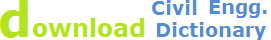# Curvature and Refraction

## Curvature of the earth:The earth appears to “fall away” with distance. The curved shape of the earth means that the level surface through the telescope will depart from the horizontal plane through the telescope as the line of sight proceeds to the horizon.

This effect makes actual level rod readings too large by:where D is the sight distance in thousands of feet.

### Effects of Curvature are:

• Rod reading is too high
• Error increases exponentially with distance

## Atmospheric Refraction:Refraction is largely a function of atmospheric pressure and temperature gradients, which may cause:

the bending to be up or down by extremely variable amounts.

There are basically three types of temperature gradient (dT/dh):

1. Absorption: occurs mainly at night when the colder ground absorbs heat from the atmosphere.

• This causes the atmospheric temperature to increase with distance from the ground and dT/dh > 0.

2. Emission: occurs mainly during the day when the warmer ground emits heat into the atmosphere, resulting in a negative temperature gradient, i.e. dT/dh < 0.

3. Equilibrium: no heat transfer takes place (dT/dh = 0) and occurs only briefly in the evening and morning.

4. The result of dT/dh < 0 is to cause the light ray to be convex to the ground rather than concave as generally shown.

• This effect increases the closer to the ground the light ray gets and errors in the region of 5 mm/km have resulted.

The atmosphere refracts the horizontal line of sight downward, making the level rod reading smaller. The typical effect of refraction is equal to about 14% of the effect of earth curvature.

## Combined Effect of Curvature and Refraction in Survey

The combined effect of curvature and refraction is approximatelyThe formula for computing the combined effect of curvature and refraction is:

C + R = 0.021K2

Where C = correction for curvature

R = correction for refraction

K = sighting distance in thousands of feet

## Correlations for various distances

 Distance Correction 100' 0.00021' 200' 0.00082' 500' 0.0052' 700' 0.01' 1 mile 0.574'

## How to eliminate error due to Curvature and Refraction

1. Proper field procedures (taking shorter shots and balancing shots) can practically reduce errors

2. Wherever possible, staff readings should be kept at least 0.5 m above the ground,

3. Using short observation distances (25 m) equalized for backsight and foresight

4. Air below is denser than air above Air below is denser than air above, Line of sight is bent downward which Negates earth curvature error by 14%.

5. Simultaneous Reciprocal Trigonometrical Heighting

6. Observations made at each station at exactly the same time, cancels the effects of curvature and refraction

If you haven't found what you were looking for on this page, fill the form below to tell us what you need to know earth Curvature & Refraction

 Related Pages Related pages Baseline measurement and Setting Out curves Triangular system of soundings Compound curvesCourse outlinesAbout Us | Site Map | Privacy Policy | Contact Us | © 2014 WebTechTix